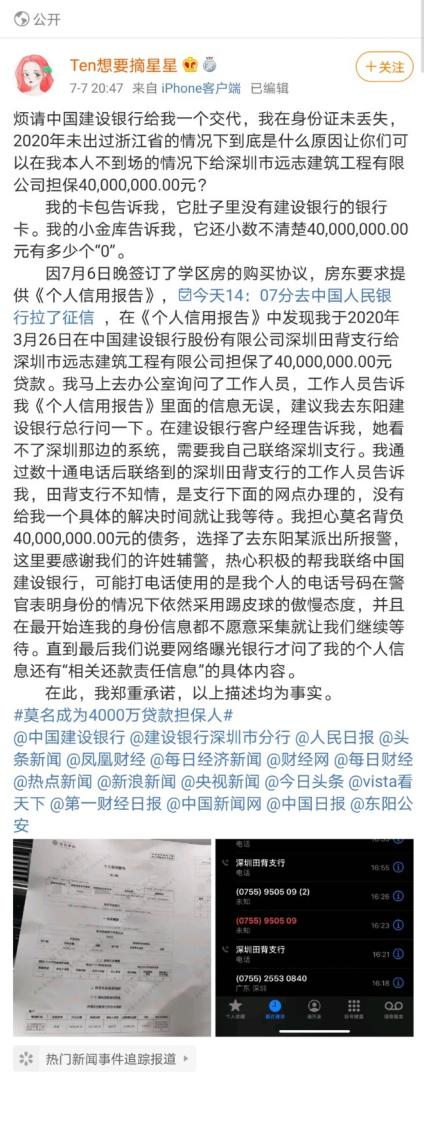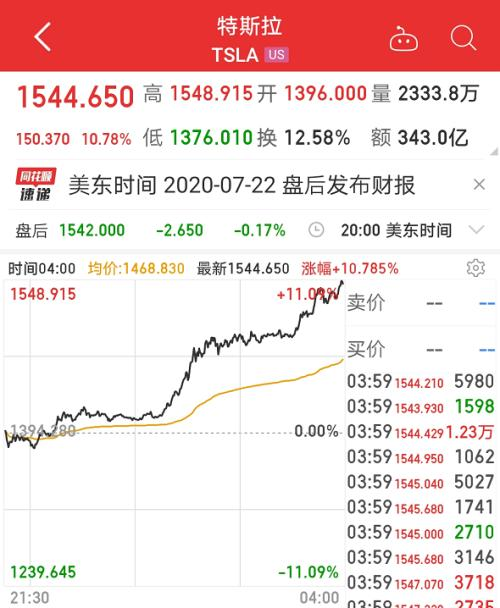## 头条4000万从天而降 不是外快而是债！小心银行神操作不是第一次

• 国内财经
• 国外财经
• 产经新闻推荐

## 4000万从天而降 不是外快而是债！小心银行神操作不是第一次推荐

## 干实业的打败搞金融的？身家一夜暴增60亿美元！马斯克超巴菲特 成全球第七大富豪推荐

## 360应邀出席2020数字金融峰会 共议新环境下的金融行业安全挑战

• 通达信公式
• 大智慧公式
• 同花顺公式
• 飞狐公式
•## 通达信散户线+黑马出击指标公式

LC:=REF(CLOSE,1);RSI5:=((SMA(MAX((CLOSE - LC),0),5,1) / SMA(ABS((CLOSE - LC)),5,1)) * 100);TR1:=SUM(MAX(MAX((HIGH - LOW),ABS((HIGH - REF(CLOSE,1)))),ABS((LOW - REF(CLOSE,1)))),10);HD:=(HIGH - REF(HIGH,1));LD:=(REF(LOW,1) - LOW);DMP:=SUM(IF(((HD > 0) AND (HD > LD)),HD,0),10);DMM:=SUM(IF(((LD > 0) AND (LD > HD)),LD,0),10);PDI:=((DMP * 100) / TR1);MDI:=((DMM * 100) / TR1);ADX:=MA(((ABS((MDI - PDI)) / (MDI + PDI)) * 100),5);AV:=(RSI5 + ADX);DXR:=(((ADX + REF(ADX,5)) / 2) + RSI5);0,COLORFF00FF;WR10:=((100 * (HHV(HIGH,10) - CLOSE)) / (HHV(HIGH,10) - LLV(LOW,10)));NEWVOL:=(RSI5 - WR10);阶段底部:=(AV + NEWVOL);STICKLINE((阶段底部 < 0),0,-10,0.8,1),LINETHICK6,COLORYELLOW;趋势线:=LLV(阶段底部,1),COLORWHITE,LINETHICK0;STICKLINE(CROSS(阶段底部,0),0,-10,0.8,1),LINETHICK6,COLORRED;STICKLINE((趋势线 >230 ),100,90,0.3,1),LINETHICK6,COLORGREEN;LOWV:=LLV(LOW,9);HIGHV:=HHV(HIGH,9);RSV:=EMA((CLOSE-LOWV)/(HIGHV-LOWV)*100,3);K:EMA(RSV,3);D:MA(K,3);STICKLINE(K>D , K,D ,2.5,0),COLORRED,LINETHICK2;STICKLINE(D>K,K,D,2.5,0),COLORGREEN,LINETHICK2;逃顶线:90,COLORGREEN;中轴线: 50,COLORRED;抄底线:10,COLORGREEN;DRAWICON(CROSS(K,D),12,1)COLORRED;DRAWICON(CROSS(D,K),88,2)COLORGREEN;警戒:阶段底部 < 0,LINETHICK0,COLORYELLOW;出击:CROSS(阶段底部,0),LINETHICK0,COLORRED;AA1:=(CLOSE-LLV(LOW,9))/(HHV(HIGH,9)-LLV(LOW,9))*200-100;AA2:=SMA(AA1,3,1);AA3:=SMA(AA2,3,1);AA4:=(3*AA2-2*AA3)/5+50;AA5:=DRAWLINE(AA4REF(AA4,1),AA4,0);AA6:=AA4>REF(AA4,1);AA7:=DRAWLINE(AA6,AA4,AA4REF(1.5*(BB3-BB4),1);STICKLINE(BB5,0,AA5,0,-1),COLOR77FFFF;STICKLINE(AA40,4,-1,5,0),COLORBBBBBB;通达信散户线+黑马出击指标公式的选股公式如下：http://www.gupang.com/202007/52168.html

•## 通达信不错的KDJ指标公式

STICKLINE(1,0,30,10,0)COLOR003300,LINETHICK2;STICKLINE(1,70,100,10,0)COLOR000033,LINETHICK2;STICKLINE(1,70,30,10,0)COLOR333333,LINETHICK2;RSV:=(CLOSE-LLV(LOW,9))/(HHV(HIGH,9)-LLV(LOW,9))*100;K:SMA(RSV,3,1);D:SMA(K,3,1);J:3*K-2*D;{DRAWICON(CROSS(J,D),0,1);}DRAWICON(CROSS(D,J),100,2);PLOYLINE((J>100),100),COLORRED,LINETHICK2;超跌:PLOYLINE((J>0),0),COLORGREEN,LINETHICK2;IF(1 ,CONST(J),DRAWNULL),COLOR009999,LINETHICK1;IF(J>90 ,J,DRAWNULL),COLOR00FFFF,LINETHICK3;IF(J

•## 通达信初步筛选指标公式

N:=20;F:=50;T:=250;ZF:=(C/REF(C,N)-1)*100>F;XG:EXIST(ZF,T)>0;通达信初步筛选指标公式的选股公式如下：

•## 通达信强势横盘不破选股指标公式

ZT:=C>=ZTPRICE(REF(C,1),0.1) AND C=H;TJ1:=HHV(H,BARSLAST(ZT))=MIN(REF(O,BARSLAST(ZT)),REF(C,BARSLAST(ZT)+1));TJ1 AND TJ2 AND BARSLAST(ZT)>2;通达信强势横盘不破选股指标公式的选股公式如下：

•## 通达信突破炸板选股指标公式

ZB:=H=ZTPRICE(REF(C,1),0.1) AND C

•## 通达信严重超买超卖指标公式

严重超买:100,COLORRED,DOTLINE;超买:80,COLORRED,DOTLINE;超卖:20,COLOR00FF00,DOTLINE;严重超卖:0,COLOR00FF00,DOTLINE;VAR1:=((CLOSE - LLV(LOW,15))/(HHV(HIGH,15) - LLV(LOW,15)))*(100);VAR2:=REVERSE(VAR1);VAR3:=SMA(VAR1,5,1);K:=SMA(VAR3,3,1);D:=SMA(K,3,1);STICKLINE((K >= D),K,D,1,0),COLORRED;STICKLINE((K = D8),K8,D8,1,0),COLORMAGENTA;STICKLINE((K8 = D88),K88,D88,1,0),COLOR0080FF;STICKLINE((K88

•## 通达信天机筹码指标公式

获利筹码:EMA(WINNER(C)*70,5),COLORRED;亏损筹码:EMA((WINNER(C*1.1)-WINNER(C*0.9))*80,5),COLORF0FF00;STICKLINE(亏损筹码>0,0,亏损筹码,5,0),COLOR66FF00;STICKLINE(亏损筹码>0,0,亏损筹码*0.9,5,0),COLOR66EE00;STICKLINE(亏损筹码>0,0,亏损筹码*0.85,5,0),COLOR66DD00;STICKLINE(亏损筹码>0,0,亏损筹码*0.8,5,0),COLOR66CC00;STICKLINE(亏损筹码>0,0,亏损筹码*0.75,5,0),COLOR66BB00;STICKLINE(亏损筹码>0,0,亏损筹码*0.7,5,0),COLOR66AA00;STICKLINE(亏损筹码>0,0,亏损筹码*0.65,5,0),COLOR669900;STICKLINE(亏损筹码>0,0,亏损筹码*0.6,5,0),COLOR668800;STICKLINE(亏损筹码>0,0,亏损筹码*0.55,5,0),COLOR667700;STICKLINE(亏损筹码>0,0,亏损筹码*0.5,5,0),COLOR666600;STICKLINE(亏损筹码>0,0,亏损筹码*0.45,5,0),COLOR665500;STICKLINE(亏损筹码>0,0,亏损筹码*0.4,5,0),COLOR664400;STICKLINE(亏损筹码>0,0,亏损筹码*0.35,5,0),COLOR663300;STICKLINE(亏损筹码>0,0,亏损筹码*0.3,5,0),COLOR662200;STICKLINE(亏损筹码>0,0,亏损筹码*0.25,5,0),COLOR661100;STICKLINE(亏损筹码>0,0,亏损筹码*0.2,5,0),COLOR660000;主力控筹:IF(获利筹码>亏损筹码,获利筹码*2,获利筹码),COLORRED;STICKLINE(主力控筹>0,0,主力控筹,5,0),COLORFFFFFF;STICKLINE(主力控筹>0,0,主力控筹*0.9,5,0),COLOREEEEEE;STICKLINE(主力控筹>0,0,主力控筹*0.85,5,0),COLORDDDDDD;STICKLINE(主力控筹>0,0,主力控筹*0.8,5,0),COLORCCCCCC;STICKLINE(主力控筹>0,0,主力控筹*0.75,5,0),COLORBBBBBB;STICKLINE(主力控筹>0,0,主力控筹*0.7,5,0),COLORAAAAAA;STICKLINE(主力控筹>0,0,主力控筹*0.65,5,0),COLOR999999;STICKLINE(主力控筹>0,0,主力控筹*0.6,5,0),COLOR888888;STICKLINE(主力控筹>0,0,主力控筹*0.55,5,0),COLOR777777;STICKLINE(主力控筹>0,0,主力控筹*0.5,5,0),COLOR666666;STICKLINE(主力控筹>0,0,主力控筹*0.45,5,0),COLOR555555;STICKLINE(主力控筹>0,0,主力控筹*0.4,5,0),COLOR444444;STICKLINE(主力控筹>0,0,主力控筹*0.35,5,0),COLOR333333;STICKLINE(主力控筹>0,0,主力控筹*0.3,5,0),COLOR222222;STICKLINE(主力控筹>0,0,主力控筹*0.25,5,0),COLOR111111;STICKLINE(主力控筹>0,0,主力控筹*0.2,5,0),COLOR000000;STICKLINE(获利筹码>0,0,获利筹码,5,0),COLORFFFFFF;STICKLINE(获利筹码>0,0,获利筹码*0.9,5,0),COLOREEEEFF;STICKLINE(获利筹码>0,0,获利筹码*0.85,5,0),COLORDDDDFF;STICKLINE(获利筹码>0,0,获利筹码*0.8,5,0),COLORCCCCFF;STICKLINE(获利筹码>0,0,获利筹码*0.75,5,0),COLORBBBBFF;STICKLINE(获利筹码>0,0,获利筹码*0.7,5,0),COLORAAAAFF;STICKLINE(获利筹码>0,0,获利筹码*0.65,5,0),COLOR9999FF;STICKLINE(获利筹码>0,0,获利筹码*0.6,5,0),COLOR8888FF;STICKLINE(获利筹码>0,0,获利筹码*0.55,5,0),COLOR7777FF;STICKLINE(获利筹码>0,0,获利筹码*0.5,5,0),COLOR6666FF;STICKLINE(获利筹码>0,0,获利筹码*0.45,5,0),COLOR5555FF;STICKLINE(获利筹码>0,0,获利筹码*0.4,5,0),COLOR4444FF;STICKLINE(获利筹码>0,0,获利筹码*0.35,5,0),COLOR3333FF;STICKLINE(获利筹码>0,0,获利筹码*0.3,5,0),COLOR2222FF;STICKLINE(获利筹码>0,0,获利筹码*0.25,5,0),COLOR1111FF;STICKLINE(获利筹码>0,0,获利筹码*0.2,5,0),COLOR0000FF;AI:=AMOUNT/VOL/100;C0:=REF(C,2)*0.865;C1:=REF(C,13)*0.772;DSY:=100*VOL/CAPITAL;抄底:IF((C-MIN(C0,C1))/C小股民筹码, 主力筹码,小股民筹码,5,0),COLOR0AF5EF;STICKLINE(主力筹码

•## 通达信净利润断层选股指标公式

N:=10;A1:=(C>=ZTPRICE(REF(CLOSE,1),0.1))&&LOW>(REF(HIGH,1)+0.001);A2:=BARSLAST(A1);A3:=EVERY(L>REF(H,A2+1),A2);EXIST(A1,N) AND A3;通达信净利润断层选股指标公式的选股公式如下：

•## 大智慧个股热度指标公式

a1:=ema(c,1);a2:=ema(c,5);a3:=ema(c,10);a4:=ema(c,20);a5:=ema(c,30);a6:=ema(c,60);a7:=if(a1-a2>=0,1,0);a8:=if(a1-a3>=0,1,0);a9:=if(a1-a4>=0,1,0);a10:=if(a1-a5>=0,1,0);a11:=if(a1-a6>=0,1,0);a12:=(a7+a8+a9+a10+a11);短线:a1;中线:a6;热度:a12,linethick0;partline(短线,a12=0,rgb(0,102,0),a12=1,rgb(0,200,0),a12=2,rgb(0,244,0),a12=3,rgb(244,244,0),a12=4,rgb(255,102,0),a12=5,rgb(220,20,60));partline(中线,a12=0,rgb(0,102,0),a12=1,rgb(0,200,0),a12=2,rgb(0,244,0),a12=3,rgb(0,244,0),a12=4,rgb(244,244,0),a12=5,rgb(220,20,60));a13:=短线-中线;stickline(a12=0 and 短线>=中线,中线*1.01,短线*0.99,5,0),color006600;stickline(a12=0 and 短线=中线,中线*1.01,短线-a13*0.47,5,0),color00c800;stickline(a12=1 and 短线>=中线,短线-a13*0.53,短线*0.99,5,0),color006600;stickline(a12=1 and 短线=中线,短线-a13*0.53,短线*0.99,5,0),color00c800;stickline(a12=2 and 短线=中线,短线-a13*0.53,短线*0.99,5,0),color00f400;stickline(a12=3 and 短线=中线,短线-a13*0.53,短线*0.99,5,0),coloryellow;stickline(a12=4 and 短线

•## 大智慧九转序列主图指标公式

a:="STSFunc@CALSTSL";R1:="STSFunc@STS_RISK1";R2:="STSFunc@STS_RISK2";S1:="STSFunc@STS_STS1";S2:="STSFunc@STS_STS2";TBS:="STSFunc@STS_STSBS";DN:="STSFunc@STS_STSDN";UP:="STSFunc@STS_STSUP"; drawtext(S1>0,L,STR(S1)); drawtext(S1

•## 大智慧资金流动曲线指标公式

VAR1:=AMOUNT/VOL/100; VAR2:=(3*HIGH+LOW+OPEN+2*CLOSE)/7;金额:SUM(AMOUNT,34)/VAR1/100,LINETHICK0,Color9999FF;VAR4:=DMA(VAR2,VOL/金额); VAR5:=100*(C-VAR4)/VAR4; 强:(MA(VAR5,20)+STDP(VAR5,20)),COLORFF00FF,LINETHICK0;弱:(MA(VAR5,20)-STDP(VAR5,20)),COLOR00FF00,LINETHICK0; 强弱线:(强+弱)/2,POINTDOT,ColorCD5A6A; PARTLINE(强弱线,(强弱线>REF(强弱线,1))-1,rgb(0,255,0),1,rgb(255,0,0) ),LINETHICK2,POINTDOT;資金差:VAR5,LINETHICK2,color0045FF;流入:CROSS(資金差,强弱线),LINETHICK0,COLORRED;DRAWICON((CROSS(資金差,强弱线)),强弱线,9);DD:=(資金差>REF(資金差,1));趋向:cross(DD,0) and 資金差-26,LINETHICK0,COLOR00FF00;DRAWICON((CROSS(dd,0)) and 資金差

•## 大智慧三线通气主图指标公式

均价:=(2*C+(C+H+L+O)/4+O)/4;R:=(3*L+2*均价)/5;R1:=(3*H+2*均价)/5;kX:=(R+R1)/2;a:=eMA(SUM(KX*V,3)/SUM(V,3),13);b:=(EMA((COST(77)),13)+A)/2;CCC:=ABS(kx-eMA(a,13))/eMA(a,13); aj:DMA(kx,CCC);CC:=ABS(a-MA(b,13))/MA(b,13); xS:DMA(A,CC);oq:(AJ+xs)/2; 大智慧三线通气主图指标公式的选股公式如下：

•## 大智慧实用短线抢筹指标公式

CJ:=(H+L+2*O+3*C)/7;线:SMA((CLOSE-LLV(LOW,11))/(HHV(HIGH,11)-LLV(LOW,11))*100,3,1),colorffffff;DK:=INTPART(276/POW(DATATYPE+0.3,2)-1.1);DN:=if(DK=0,slope(短筹,0.4*DN)*POW(0.61*DN,1.5),0);活筹:=0.28*短筹+4.62*抢筹-3;TT:=(HHV(atan(slope(活筹,0.6*DN)*0.58)*180/3.14159,0.6*DN)+1.11*短筹)/1.916;屯:=SMA(TT,2.4*DN,0.6*DN);屯筹:(2.28*HHV(屯,1.6*DN)+SMA(屯,2.4*DN,1))/2.801,COLORFFff00;PARTLINE(屯筹,slope(屯筹,0.4*DN)>0),colorred,linethick3;PARTLINE(屯筹,slope(屯筹,0.4*DN)0,0.22*SMA(QL,2.4*DN,2.4*DN-1)+0.44*HHV(QL,1.6*DN)-4,0);潜伏期:=BARSLAST(cross(40,潜筹));DRAWICON(CROSS(线,50),47,10);DRAWICON(屯筹70 AND 线>88,105,11);DRAWICON(CROSS(屯,短筹) AND 短筹REF(潜伏期,1),4)=4 AND 潜筹10 AND 屯筹>REF(屯筹,1),5,8);V1:=(HHV(HIGH,5)-LLV(LOW,5));V2:=(HHV(HIGH,9)-CLOSE);V3:=(CLOSE-LLV(LOW,9));V4:=V2/V1*100-70 ;V5:=(CLOSE-LLV(LOW,60))/(HHV(HIGH,60)-LLV(LOW,60))*100;V6:=(2*C+H+L)/4;V7:=SMA((V3/V1*100),3,1); V8:=LLV(LOW,34);V9:=SMA(V7,3,1)-SMA(V4,9,1);V10:= IF(V9>100,V9-100,0);VA:=HHV(HIGH,34);中线涨:STICKLINE(EMA((V6-V8)/(VA-V8)*100,8)>0 AND EMA((V6-V8)/(VA-V8)*100,8)-EMA(EMA((V6-V8)/(VA-V8)*100,8),5)>=0,49,50,15,0),Color4080FF,linethick6;中线跌:STICKLINE(EMA((V6-V8)/(VA-V8)*100,8)>0 AND EMA((V6-V8)/(VA-V8)*100,8)-EMA(EMA((V6-V8)/(VA-V8)*100,8),5)

•## 大智慧完美动量线指标公式

量:=V;换手:V/CAPITAL*100,LINETHICK0;VAR1:=VOL/((H-L)*2-ABS(C-O));买盘:=IF(C>O,VAR1*(H-L),IF(CO,0-VAR1*((H-C)+(O-L)),IF(CREF(DLX3,1),DLX3),LINETHICK2,Color00FFFF;partline(DLX30,SUM(AMOUNT,A1)/SUM(VOL,A1)/100,EMA(CLOSE,120));百分之百换手价:CMA,LINETHICK0,ColorFFC0FF;{100%换手平均成本价}; 大智慧完美动量线指标公式的选股公式如下：

•## 大智慧王者通道主图指标公式

M:=33;EMA10:MA(C,10) ,COLORBLUE, LINETHICK2;TY:=DYNAINFO(8)>0;GG:=V=LLV(V,100) AND TY;DRAWICON(GG,LOW*0.96,1);DRAWICON(GG,LOW*0.95,17);现价:CLOSE,POINTDOT,COLOR000F89;均价:=(2*C+(H-L)/2+L+O)/4;A:MA(HHV(均价,8),8),COLORWHITE,LINETHICK2;A1:MA(A,2),COLORWHITE;A2:MA(A1,2),COLORWHITE;A3:MA(A2,2),COLORWHITE;PARTLINE(A,A>REF(A,1),RGB(255,0,0)),LINETHICK2;PARTLINE(A1,A1>REF(A1,1),RGB(255,0,0));PARTLINE(A2,A2>REF(A2,1),RGB(255,0,0));PARTLINE(A3,A3>REF(A3,1),RGB(255,255,0));B:MA(LLV(均价,8),8),COLORAAAA00,LINETHICK2;B1:MA(B,2),COLORGREEN;B2:MA(B1,2),COLORGREEN;B3:MA(B2,2),COLORGREEN;PARTLINE(B,B>REF(B,1),RGB(255,0,255)),LINETHICK2;PARTLINE(B1,B1>REF(B1,1),RGB(255,255,0));PARTLINE(B2,B2>REF(B2,1),RGB(255,255,0));PARTLINE(B3,B3>REF(B3,1),RGB(255,255,0)); 大智慧王者通道主图指标公式的选股公式如下：

•## 大智慧游资对倒成本指标公式

NUM:=13;SHORT:=3;LONG:=5;ZF:=H-L;CB:=AMOUNT/VOL/100;DV1:=IF(ZF>0 AND C>CB, ((H-C)/(H-CB))*((H-C)/(H-CB))*((H-CB)/ZF*VOL) ,0);UV1:=IF(ZF>0 AND C>CB, VOL-DV1 ,0);UV2:=IF(ZF>0 AND C0 AND CREF(C,1), VOL,0);DV3:=IF(ZF=0 AND C0,0,CBX,4,0),Color0984FF;STICKLINE(CBX>0,0,CBX,5,0),Color2D96FF;STICKLINE(CBX>0,0,CBX,2,0),Color53A9FF;STICKLINE(CBX>0,0,CBX,1,0),Color80BFFF;STICKLINE(CBX>0,0,CBX,0,0),ColorA4D1FF;STICKLINE(CBX

•## 同花顺跑步进场指标公式

VAR1:=REF((LOW+OPEN+CLOSE+HIGH)/4,1);VAR2:=SMA(ABS(LOW-VAR1),13,1)/SMA(MAX(LOW-VAR1,0),10,1);VAR3:=EMA(VAR2,10);VAR4:=LLV(LOW,33);VAR5:=EMA(IF(LOWREF(VAR5,1),VAR5,0),colorred,NODRAW;STICKLINE(VAR5>REF(VAR5,1),0,VAR5,3,0 ),colorred;洗盘:IF(VAR5

•## 同花顺技术抄底指标公式

VAR1:=EMA(HHV(HIGH,500),21);VAR2:=EMA(HHV(HIGH,250),21);VAR3:=EMA(HHV(HIGH,90),21);VAR4:=EMA(LLV(LOW,500),21);VAR5:=EMA(LLV(LOW,250),21);VAR6:=EMA(LLV(LOW,90),21);VAR7:=EMA((VAR4*0.96+VAR5*0.96+VAR6*0.96+VAR1*0.558+VAR2*0.558+VAR3*0.558)/6,21);VAR8:=EMA((VAR4*1.25+VAR5*1.23+VAR6*1.2+VAR1*0.55+VAR2*0.55+VAR3*0.65)/6,21);VAR9:=EMA((VAR4*1.3+VAR5*1.3+VAR6*1.3+VAR1*0.68+VAR2*0.68+VAR3*0.68)/6,21);VARA:=EMA((VAR7*3+VAR8*2+VAR9)/6*1.738,21);VARB:=REF(LOW,1);VARC:=SMA(ABS(LOW-VARB),3,1)/SMA(MAX(LOW-VARB,0),3,1)*100;VARD:=EMA(IF(CLOSE*1.350,今量*1.2,0),STICK,LINETHICK5, COLOR0000FF;A2:IF(火焰山>0,今量*0.8,0),STICK,LINETHICK5, COLOR0066FF;A3:IF(火焰山>0,今量*0.6,0),STICK,LINETHICK5, COLOR0099FF;A4:IF(火焰山>0,今量*0.4,0) ,STICK,LINETHICK5,COLOR00CCFF;A5:IF(火焰山>0,今量*0.2,0) ,STICK,LINETHICK5,COLOR00FFFF; 同花顺技术抄底指标公式的选股公式如下：

•## 同花顺KD变化指标公式

N:=5;M1:=3;M2:=3;分界:50,colorblue;RSV:=(CLOSE-LLV(LOW,N))/(HHV(HIGH,N)-LLV(LOW,N))*100;K:SMA(RSV,M1,1);D:SMA(K,M2,1);Bar:=(K-D)*2;STICKLINE(Bar>=0,Bar,0,1,0),RGB(255,0,0);STICKLINE(Bar

•## 同花顺魔法CCI指标公式

TYP:=(HIGH+LOW+CLOSE)/3;经典CCI战法:EMA((TYP-MA(TYP,36.0270012))/(0.015*AVEDEV(TYP,36.0270012)),2.0332),colorwhite;STICKLINE(经典CCI战法0,-150,-151,2,0),colorblue;MA20:=MA(C,20);MA60:=MA(C,60);MA120:=MA(C,120);角度MA20:=ATAN((MA20/REF(MA20,1)-1)*100)*180/3.1416;角度MA60:=ATAN((MA60/REF(MA60,1)-1)*100)*180/3.1416;角度MA120:=ATAN((MA120/REF(MA120,1)-1)*100)*180/3.1416;DRAWICON(MA20>MA60 AND MA60>MA120 AND 角度MA60>0 AND 角度MA120>0 AND (C-L)/C*100 >=5 AND 经典CCI战法-150 ,LOW,13); 同花顺魔法CCI指标公式的选股公式如下：

•## 同花顺低吸成金指标公式

FSHORT:=21;FLONG:=5;多空线:EMA(CLOSE,FLONG),colorwhite,LINETHICK2;操盘线:=EMA(CLOSE,FSHORT);多线:IFF(操盘线>多空线,操盘线 ,DRAWNULL),colorgreen,LINETHICK2;空线:IFF(操盘线=REF(多空线,1) AND 操盘线

•## 同花顺免费DDX大单指标公式

IF(PERIODNAME"日线"){统计:"该指标只在日线周期下有效。";RETURN;}r1:=ZDMR[-1];r2:=BDMR[-1];r3:=-ZDMC[-1];r4:=-BDMC[-1];x1:=(ZDMR+BDMR);y1:=-(ZDMC+BDMC);STICKLINE(x1+y1>=0,5*(x1+y1),0,8,0),colorred;STICKLINE(x1+y1DDX2,RGB(255,0,0),DDX1b3){y=a3-b3;z=y/VOL1*100;统计:TOSTRING(1,0)+"天机构买入"+TOSTRING(a3,1)+"万手,卖出"+TOSTRING(b3,1)+"万手.净买入"+TOSTRING(y,1)+"万手.占总成交"+TOSTRING(z,1)+"％.";}ELSE{y=b3-a3;z=y/VOL1*100;统计:TOSTRING(1,0)+"天机构买入"+TOSTRING(a3,1)+"万手,卖出"+TOSTRING(b3,1)+"万手.净卖出"+TOSTRING(y,1)+"万手.占总成交"+TOSTRING(z,1)+"％.";} 同花顺免费DDX大单指标公式的选股公式如下：

•## 同花顺铁锅MACD指标公式

SHORT:=13;LONG:=77;M:=13;DIFF : EMA(CLOSE,SHORT) - EMA(CLOSE,LONG);DEA : EMA(DIFF,M);MACD : 2*(DIFF-DEA/2);Zero : 0;STICKLINE(MACD>0,MACD,0,1,1),COLOR0000AA;STICKLINE(MACDREF(DIFF,1),RGB(255,0,0),DIFFREF(DEA,1),RGB(255,0,0),DEA

•## 同花顺异动反转指标公式

N:=9;M1:=3;M2:=3;RSV:=(CLOSE-LLV(LOW,N))/(HHV(HIGH,N)-LLV(LOW,N))*100;K:=SMA(RSV,M1,1);D:=SMA(K,M2,1);J:=3*K-2*D;VAR2:=REF(LOW,1);VAR3:=SMA(ABS(LOW-VAR2),3,1)/SMA(MAX(LOW-VAR2,0),3,1)*100;VAR4:=EMA(VAR3*10,3);VAR5:=LLV(LOW,13);VAR6:=HHV(VAR4,13);VAR7:=EMA(IF(LOW500,500,VAR7);STICKLINE(VAR8>-120,0,VAR8,9,1),colorgray;STICKLINE(VAR8>1 AND J>REF(J,1) AND REF(J,1)0.1 AND VAR8REF(J,1) AND REF(J,1)0.01 AND "J"

•## 飞狐主力净买量指标公式

A:=VOL*CLOSE;B:=SUM(A,2)/SUM(VOL,2);B1:=EMA(B,7);M:=(B-B1);STICKLINE(M>0,0,M,2,0),COLORRED;STICKLINE(M

•## 飞狐MACD和KDJ主图指标公式

INPUT:N(18),S(26),P(12),M(9);DRAWGBK(CLOSE=CLOSE,COLORRGB(80,130,130),COLORRGB(30,30,60),1);A:=EMA(CLOSE,S);T:=EMA(CLOSE,P);B:=EMA(A,M);T1:=EMA(T,M);DEA:=EMA(T-(T1-T),2),COLORFF8866,LINETHICK2;DIFF:=DEA-EMA(((A-B)-(T-T1)),1)*6,COLORFFFF66,LINETHICK2;FILLRGN(DIFF>DEA,DEA,DIFF),COLORBROWN;FILLRGN(DIFFOPEN or CLOSE=OPEN and CLOSE>REF(CLOSE,1),LOW,HIGH,0.1,0),colorred;STICKLINE(CLOSE>OPEN or CLOSE=OPEN and CLOSE>REF(CLOSE,1),OPEN,CLOSE,4,0),colorred;STICKLINE(CLOSE=CLOSE ,CLOSE ,OPEN,3,0 ),COLOR00AA00;STICKLINE(OPEN>=CLOSE ,CLOSE ,OPEN,2.5,0 ),COLOR00BB00;STICKLINE(OPEN>=CLOSE ,CLOSE ,OPEN,2,0 ),COLOR00CC00;STICKLINE(OPEN>=CLOSE ,CLOSE ,OPEN,1.5,0 ),COLOR00DD00;STICKLINE(OPEN>=CLOSE ,CLOSE ,OPEN,1,0 ),COLOR00EE00;STICKLINE(OPEN>=CLOSE ,CLOSE ,OPEN,0.5,0 ),COLOR00FF00;STICKLINE(CLOSE>OPEN,CLOSE,OPEN,0,0) ,COLORFFBBFF;STICKLINE(CLOSE>OPEN,CLOSE,OPEN,4,0) ,COLORFF00FF;STICKLINE(CLOSE>OPEN,CLOSE,OPEN,3.5,0) ,COLORFF22FF;STICKLINE(CLOSE>OPEN,CLOSE,OPEN,3,0) ,COLORFF44FF;STICKLINE(CLOSE>OPEN,CLOSE,OPEN,2.5,0) ,COLORFF66FF;STICKLINE(CLOSE>OPEN,CLOSE,OPEN,2,0) ,COLORFF88FF;STICKLINE(CLOSE>OPEN,CLOSE,OPEN,1.5,0) ,COLORFF99FF;STICKLINE(CLOSE>OPEN,CLOSE,OPEN,1,0) ,COLORFFAAFF;STICKLINE(CLOSE>OPEN,CLOSE,OPEN,0.5,0) ,COLORFFBBFF;{涨跌停}ZDF:=(C-REF(C,1))/REF(C,1)*100;{STICKLINE(ZDF>9.8,O,C,4,0),COLORFF00FF;STICKLINE(ZDF9.8,CLOSE,O,2.8,0),COLOR000055;STICKLINE(ZDF>9.8,CLOSE,O,2,0),COLOR000088;STICKLINE(ZDF>9.8,CLOSE,O,1.5,0),COLOR0000AA;STICKLINE(ZDF>9.8,CLOSE,O,1,0),COLOR0000CC;STICKLINE(ZDF>9.8,CLOSE,O,0.5,0),COLOR0000EE;STICKLINE(ZDF

•## 飞狐KDJ共振指标公式

月K:"KDJ.K#MONTH"(9,3,3),COLOR0080FF;月D:"KDJ.D#MONTH"(9,3,3),COLOR0080FF,LINETHICK3;月J:="KDJ.J#MONTH"(9,3,3);周K:"KDJ.K#WEEK"(9,3,3),COLORRED;周D:"KDJ.D#WEEK"(9,3,3),COLORRED,LINETHICK3;周J:="KDJ.J#WEEK"(9,3,3);日K:"KDJ.K"(9,3,3),COLORWHITE;日D:"KDJ.D"(9,3,3),COLORWHITE,LINETHICK3;日J:="KDJ.J"(9,3,3);日金叉:IF(CROSS(日K,日D) AND (日D51),20,0),COLORWHITE;周金叉:IF(CROSS(周K,周D) AND (周D51),30,0),COLORRED;月金叉:IF(CROSS(月K,月D) AND (月D51),40,0),COLOR0080FF;共振:(周金叉 AND 日金叉) OR (周金叉 AND 月金叉) OR (日金叉 AND 月金叉),COLORYELLOW;STICKLINE(共振,0,50,1,0),COLORYELLOW;STICKLINE(月J100,100,月J,2,-1),COLOR0080FF;STICKLINE(周J100,100,周J,1,-1),COLORRED;STICKLINE(日J100,100,日J,0,0),COLORWHITE; 飞狐KDJ共振指标公式的选股公式如下：

•## 飞狐胡子MACD指标公式

DRAWGBK(C>1, COLORRGB(50,80,40), COLORRGB(20,70,110),1);0,COLORFF6633;DIFF:EMA(CLOSE,12) - EMA(CLOSE,26),COLORMAGENTA,LINETHICK1;DEA:EMA(DIFF,9),COLORYELLOW,LINETHICK1;MACD:2*(DIFF-DEA), COLORSTICK;MACD1:2*(DIFF-DEA),COLORRED;MACD2:WMA(MACD1,3),COLORCYAN;STICKLINE(MACD1>MACD2 AND MACD1>0,0,MACD1,2,0),COLORRED;STICKLINE(MACD1>MACD2 AND MACD1

•## 飞狐水纹波主图指标公式

G_x1:=(2*C+O+L+H)/5; MA5:= EMA(FORCAST(ma(G_x1,2),2),5);{水纹波}PARTLINE(C,REF(MA5,13)),COLORFFC010; PARTLINE(C,REF(MA5,12)),COLORFFC010; PARTLINE(C,REF(MA5,11)),COLORFFC010;PARTLINE(C,REF(MA5,10)),COLORFFC010; PARTLINE(C,REF(MA5,9)),COLORFFC010; PARTLINE(C,REF(MA5,8)),COLORFFC010;PARTLINE(C,REF(MA5,7)),COLORFFC010; PARTLINE(C,REF(MA5,6)),COLORFFC010; PARTLINE(C,REF(MA5,5)),COLORFFC010;PARTLINE(C,REF(MA5,4)),COLORFFC010; PARTLINE(C,REF(MA5,3)),COLORFFC010; PARTLINE(C,REF(MA5,2)),COLORFFC010;PARTLINE(C,REF(MA5,1)),COLORFFC010;KLINE(o,h,l,c,0); 飞狐水纹波主图指标公式的选股公式如下：

•## 飞狐周日MACD指标公式

上市日期年:REF(YEAR,BARSCOUNT(C)-1),linethick0,COLORRED;月:REF(MONTH,BARSCOUNT(C)-1),linethick0,COLORYELLOW;日:REF(DAY,BARSCOUNT(C)-1),linethick0,COLORCYAN;DIFF :=( EMA(CLOSE,12) - EMA(CLOSE,26))*2;DEA := EMA(DIFF,9);MACD := 2*(DIFF-DEA), COLORSTICK;DIFFA := "macd.diff#week";DEAA := "macd.dea#week";FILLRGN(DIFFA>DEAA,DIFFA,DEAA),COLOR3232F0;FILLRGN(DIFFAREF(DIFF,1),DIFF),linethick2,colorred;PARTLINE(DIFFREF(DIFF,1)AND REF(DIFF,1)>0,DIFF),linethick2,colorred;PARTLINE(DEA>REF(DEA,1),DEA),coloryellow;PARTLINE(DEA=ref(MACD,1)and MACD>0,0,MACD,6,0 ),COLOR0000AA;STICKLINE(MACD>=ref(MACD,1)and MACD>0,0,MACD,6.6 ,0 ),COLOR0011BB;STICKLINE(MACD>=ref(MACD,1)and MACD>0,0,MACD,4.4,0 ),COLOr0022CC;STICKLINE(MACD>=ref(MACD,1)and MACD>0,0,MACD,3.3 ,0 ),COLOR0033DD;STICKLINE(MACD>=ref(MACD,1)and MACD>0,0,MACD,2.2 ,0 ),COLOR0044EE;STICKLINE(MACD>=ref(MACD,1)and MACD>0,0,MACD,1.1 ,0 ),COLOR0055FF;STICKLINE(MACD>ref(MACD,1)and MACDref(MACD,1)and MACDref(MACD,1)and MACDref(MACD,1)and MACDref(MACD,1)and MACDref(MACD,1)and MACD

•## 飞狐MACD底背离找反弹指标公式

DIFF : EMA(CLOSE,12) - EMA(CLOSE,26);DEA : EMA(DIFF,9);MACD : 2*(DIFF-DEA), COLORSTICK;"macd.diff#min30",COLORRED;"macd.dea#min30",COLORGREEN; 飞狐MACD底背离找反弹指标公式的选股公式如下：

•## 飞狐轻松获利赚小钱主图指标公式

RSV:=(CLOSE-LLV(LOW,9))/(HHV(HIGH,9)-LLV(LOW,9))*100;K:=SMA(RSV,3,1);D:=SMA(K,3,1);A:=ZIG(K,40);B:=REF(ZIG(K,40),1);DRAWNUMBER(CROSS(A,B),L*0.99,C,2),COLORWHITE;DRAWNUMBER(CROSS(A,B),L*0.97,C*1.03,2),COLORRED;STICKLINE(CROSS(A,B),H,L,0,0),COLORYELLOW;STICKLINE(CROSS(A,B),C,O,8,1),COLORYELLOW;E:BARSLAST(CROSS(A,B)),LINETHICK0;F:HHV(H,6),LINETHICK0;G:REFX(F,E=5),LINETHICK0;DRAWNUMBER(E=5,F*1.03,F,2),COLORGREEN; 飞狐轻松获利赚小钱主图指标公式的选股公式如下：

• 公式技巧
• 公式教程
• 指标知识

## 热门标签

• 个人微信扫码工作时间

周一至周六 20:00-22:00

周日 07:00-22:00

• 如果你觉得本站很棒，可以通过扫码支付打赏哦！

•微信收款码
•支付宝收款码